# Ex.7.1 Q1 Cubes and Cube Roots - NCERT Maths Class 8

Go back to  'Ex.7.1'

## Question

Which of the following numbers are not perfect cubes?

(i) $$216$$

(ii) $$128$$

(iii) $$1000$$

(iv) $$100$$

(v) $$46656$$

Video Solution
Cubes And Cube Roots
Ex 7.1 | Question 1

## Text Solution

What is unknown?

To find the numbers which are not perfect cubes.

Reasoning:

A number is a prefect cube only when each factor in the prime factorization is grouped in triples.

Steps:

(i)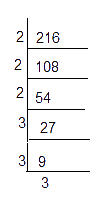\begin{align}216 &= {2 \times 2 \times 2} \times {3 \times 3 \times 3} \\&= {2^3} \times {3^3}\\& ={{(2\times 3)}^{3}} \\& ={{6}^{3}} \\\end{align}

$$\therefore 216$$ is a perfect cube

(ii)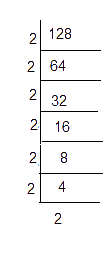\begin{align}128 = 2 \times 2 \times 2 \times 2 \times 2 \times 2 \times 2 \end{align}

$$128 = {2^3} \times {2^3} \times 2$$ one of the $$2$$ is not grouped in triples

$$\therefore \;128$$ is not a perfect cube

(iii)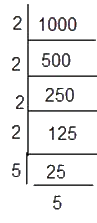\begin{align}1000 &= 2 \times 2 \times 2 \times 5 \times 5 \times 5\\ 1000 &= {2^3} \times {5^3}\end{align}

$$\therefore\;1000$$ is a perfect cube

(iv)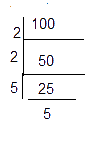\begin{align}100&= 2 \times 2 \times 5 \times 5\\&= {2^2} \times {5^2}\end{align}

Both $$2$$ and $$5$$ are not grouped in triples

$$\therefore \;100$$ is not a perfect cube.

(iv)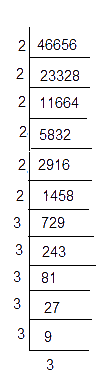\begin{align}46656 &= \left(\begin{array} \ \underline {2 \times 2 \times 2} \times \underline {2 \times 2 \times 2} \times \\ \underline {3 \times 3 \times 3} \times \underline {3 \times 3 \times 3}\end{array} \right) \\ &= {2^3} \times {2^3} \times {3^3} \times {3^3} \end{align}

$$\therefore\;46656$$ is a perfect cube

Learn from the best math teachers and top your exams

• Live one on one classroom and doubt clearing
• Practice worksheets in and after class for conceptual clarity
• Personalized curriculum to keep up with school